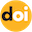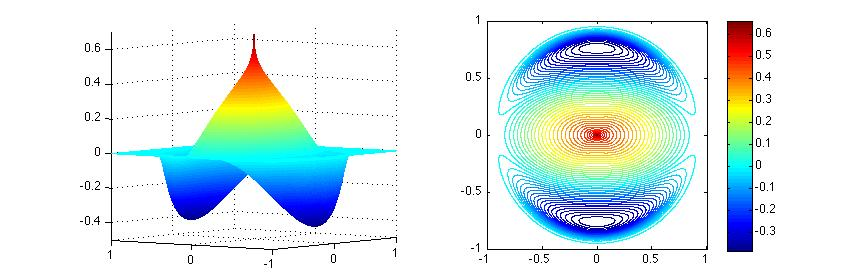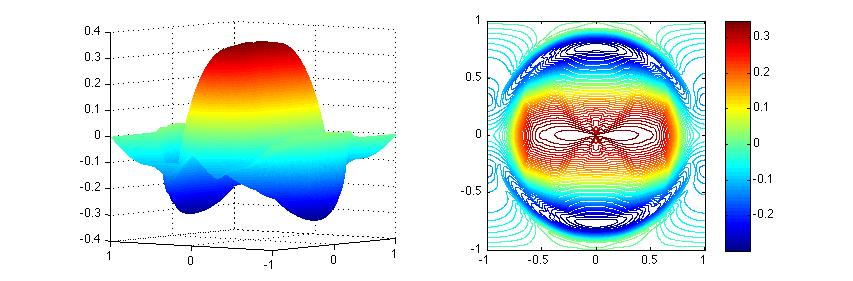Register
ResearchWednesday, 01 August 2012 11:24

## Hierarchical construction of bounded solutions to divU = F

"Nonlinear Partial Differential Equations", Proceedings of the 2010 Abel Symposium held in Oslo, Sep. 2010 (H. Holden & K. Karlsen eds.), Abel Symposia 7, Springer 2011, 255-269.

Abstract

We implement the hierarchical decomposition introduced in [Ta15], to construct uniformly bounded solutions of the problem $$\nabla\cdot U = F$$, where the two-dimensional data is in the critical regularity space, $$F\in L^2_{\#}(\mathbb{T}^2)$$. Criticality in this context, manifests itself by the lack of linear mapping, $$F\in L^2_{\#}(\mathbb{T}^2)\to U\in L^{\infty}(\mathbb{T}^2,\mathbb{R}^2)$$ [BB03]. Thus, the intriguing aspect here is that although the problem is linear, the construction of its uniformly bounded solutions is not.doi:10.1007/978-3-642-25361-4_14Download the Published Version

## Introduction

We are concerned with uniformly bounded solutions of the equation $\nabla\cdot U=F,\quad U\in L^{\infty}(\mathbb{T}^2,\mathbb{R}^2), \quad F\in L^2_{\#}(\mathbb{T}^2),$ where $$L^2_{\#}(\mathbb{T}^2)$$ is the space of $$L^2$$ integrable functions over the 2-dimensional torus $$\mathbb{T}^2$$ with zero mean.

The classical Helmholtz solution $$U_{Hel}=\nabla\Delta^{-1}F$$ is not always uniformly bounded. And what's more, we are not able to construct a solution linearly which is uniformly bounded [BB03].

Inspired by the idea of hierarchical decompositions in image processing [TNV04], Tadmor [Ta09] introduces a constructive procedure to solve the equation: the solution is given by hierarchical decompositions $$U_{Bdd}=\sum u_j$$, where the $$\{u_j\}$$'s can be computed recursively by the following minimizers, $u_{j+1} = \arg\min_{u} \left\{\|u\|_{L^{\infty}} + \lambda_1 2^j\|F-\nabla\cdot(\sum_{k=1}^j u_k) -\nabla\cdot u\|_{L^2}^2\right\}, \quad j=0,1,\cdots.$ Here $$\lambda_1$$ is a sufficiently large parameter to avoid trivial solution $$u_1\equiv 0$$.

## Analysis on the minimization problem and its dual

We rewrite each minimization step of the hierarchical decompositions in the following form, $\bar{u}=\arg\min_{u} \left\{\|u\|_{L^{\infty}}+\lambda\|f-\nabla\cdot u\|_{L^{2}}^2\right\}.$ Here, we seek a minimizer $$\bar{u}:\mathbb{T}^2\rightarrow\mathbb{R}^2$$ with $$L^{\infty}$$ norm defined as $$\|u\|_{L^{\infty}}:=\mathrm{ess } \sup_{x} \sqrt{u_1^2(x)+u_2^2(x)}$$, $$f$$ is an $$L^2$$ function with zero mean, which stands for $$\displaystyle F-\nabla\cdot(\sum_{k=1}^j u_k)$$, $$j=0,1,\cdots$$, and $$\lambda$$ is a sufficiently large parameter, which stands for the dy scale $$\lambda_1 2^j, j=0,1,\cdots$$. We also assume Neumann boundary condition for the solution $$u\cdot {\mathbf n}=0$$ for future simplicity.

To circumvent the difficulty of $$L^{\infty}$$ norm, we find the dual problem of the minimization problem $\bar{r}=\arg\inf_{r}\sup_{\mu\geq 0}\left[\lambda \left\langle r-2f,r\right\rangle +\mu\left(\|\nabla r\|_{L^1}-\frac{1}{2\lambda}\right)\right],$ where $$\bar{r}$$ is the optimal residual which satisfies $$\bar{r}=f-\nabla\cdot\bar{u}$$.

Applying minimax theorem and Euler-Lagrange equations, we have a PDE system where $$\bar{r}$$ is a solution $(r-f)-2\lambda\left\langle f-r,r\right\rangle \nabla\cdot\left(\frac{\nabla r}{|\nabla r|}\right)=0.$

Finally, $$\bar{u}$$ can be recovered by $u=2\lambda\left\langle r-f,r\right\rangle \cdot\frac{\nabla r}{|\nabla r|}.$

## Numerical implementation

We solve the problem $$\nabla\cdot U=F$$ using its hierarchical decomposition. In each iteration, we solve the minimization problem. There are 3 stages to finish one iteration.

1. Find the non-trivial solution $$r_j$$ which satisfies the PDE system with $$\lambda=\lambda_j$$ and $$f=f_j$$,
2. Recover $$u_j$$,
3. Update $$\lambda_{j+1}=2\lambda_j$$, $$f_{j+1}=r_j$$.

Initially, $$\lambda_1$$ should be greater than $$(2\|\nabla f\|_{L^1})^{-1}$$, and $$f_1=F$$. The iteration terminates when $$\|r_j\|_{L^2}$$ is sufficiently small. The final solution $$U_{Bdd}$$ is the summation of all $$u_j$$'s.

In stage 1, we solve the PDE system using the following iteration method $r^{(n+1)}_{i,j}=f_{i,j}+\frac{K(r^{(n)})}{h^2}\left[\frac{r^{(n+1)}_{i+1,j}-r^{(n+1)}_{i,j}}{\sqrt{\epsilon^2+(D_{+x}r^{(n)}_{i,j})^2+(D_{0y}r^{(n)}_{i,j})^2}}-\frac{r^{(n+1)}_{i,j}-r^{(n+1)}_{i-1,j}}{\sqrt{\epsilon^2+(D_{+x}r^{(n)}_{i-1,j})^2+(D_{0y}r^{(n)}_{i-1,j})^2}}\right]$ $+\frac{K(r^{(n)})}{h^2}\left[\frac{r^{(n+1)}_{i,j+1}-r^{(n+1)}_{i,j}}{\sqrt{\epsilon^2+(D_{0x}r^{(n)}_{i,j})^2+(D_{+y}r^{(n)}_{i,j})^2}}-\frac{r^{(n+1)}_{i,j}-r^{(n+1)}_{i,j-1}}{\sqrt{\epsilon^2+(D_{0x}r^{(n)}_{i,j-1})^2+(D_{+y}r^{(n)}_{i,j-1})^2}}\right].$ with initial $$r^{0}=f/2$$, and reflect the bounds. Here $$K(r):=2\lambda\left\langle r-f,r\right\rangle$$ and $$\epsilon$$ is a small number to avoid singularity.

In stage 2, we recover $$u$$ by $u^{1,(n+1)}_{i+1/2,j}=-\frac{K^{(n)}}{h}\cdot\frac{r^{(n+1)}_{i+1,j}-r^{(n+1)}_{i,j}}{\sqrt{\epsilon^2+(D_{+x}r^{(n)}_{i,j})^2+(D_{0y}r^{(n)}_{i,j})^2}},\quad u^{2,(n+1)}_{i,j+1/2}=-\frac{K^{(n)}}{h}\cdot\frac{r^{(n+1)}_{i,j+1}-r^{(n+1)}_{i,j}}{\sqrt{\epsilon^2+(D_{0x}r^{(n)}_{i,j})^2+(D_{+y}r^{(n)}_{i,j})^2}}.$

## Numerical Experiments

Let $$F=\Delta v$$ on $$[-1,1]\times[-1,1]$$, where $v(x,y)=\begin{cases}x\cdot|\log r|^{1/3}\cdot e^{-\frac{1}{1-r^2}}&r<1\\0&r\geq 1\end{cases},$ where $$r=\sqrt{x^2+y^2}$$.

Helmholtz solution $$U^1_{Hel}$$ is unbounded. But hierarchical solution $$U^1_{Bdd}$$ is uniformly bounded.Figure 1. Helmholtz solution $$U^1_{Hel}$$Figure 2. Hierarchical solution $$U^1_{Bdd}$$

We also introduce two steps solution $$U_{2step}=u_1+\nabla\Delta^{-1}r_1$$, where $$u_1$$ is the result of the first hierarical step, and $$r_1=F-\nabla u_1$$. $$U_{2step}$$ is also uniformly bounded.Figure 3. Two steps solution $$U^1_{2step}$$

## Resources

• Eitan Tadmor, Changhui Tan. Hierarchical construction of bounded solutions of divU = F in critical regularity spaces. "Nonlinear Partial Differential Equations", Proceedings of the 2010 Abel Symposium held in Oslo, Sep. 2010 (H. Holden & K. Karlsen eds.), Abel Symposia 7, Springer 2011, 255-269. [View PDF]

## References

• [AF03] R. Adams and J. Fournier, Sobolev Spaces, 2nd ed., Academic Press, 2003.
• [BB03] J. Bourgain and H. Brezis, On the equation $$\nabla\cdot Y=f$$ and application to control of phases, J. Amer. Math. Soc. 16 (2003), no. 2, 393--426.
• [BB07] J. Bourgain and H. Brezis, New estimates for elliptic equations and Hodge type systems, J. Eur. Math. Soc. (JEMS) 9 (2007), no. 2, 277--315.
• [BS88] C. Bennett and R. Sharpley, Interpolation of operators, Pure and Applied Mathematics, vol. 129, Academic Press, Orlando, 1988.
• [Ch04] A. Chambolle, An algorithm for total variation minimization and applications, Journal of Mathematical Imaging and Vision, vol. 20, pp. 89-97, 2004.
• [Co] A. Cohen, private communication.
• [DFT05] B. Dacorogna, N. Fusco and L. Tartar, On the solvability of the equation $$\nabla\cdot u=f$$ in $$L^1$$ and in $$C^0$$, Rend. Mat. Acc. Lincei. s. 9 v. 14 (2003), pp. 239-245.
• [ET99] I. Ekeland and R. Temam, Convex Analysis and Variational Problems, SIAM, 1999.
• [Ev10] L. Evans, Partial Differential Equations, 2nd ed., American Mathematical Society, 2010.
• [Ma06] Y. Maday, $$L^{\infty}$$-stable approximation of a solution to $$\nabla\cdot Y=f$$ for $$f\in L^2$$ in two dimensions, J. Sci. Comput. 28(2-3) (2006), pp. 451-458.
• [ON63] R. O'Neil, Convolution operators and $$L^{p,q}$$ spaces, Duke Math, J. 30, 129-143, 1963.
• [ROF92] L. Rudin, S. Osher and E. Fatemi, Nonlinear Total Variation Based Noise Removal Algorithms, Physica D, 60, pp. 259-268, 1992.
• [Ta89] L. Tartar, Lorentz spaces and applications, Lecture notes, Carnegie Mellon University, 1989.
• [Ta15] E. Tadmor, Hierarchical construction of bounded solutions in critical regularity spaces, Communications on Pure and Applied Mathematics, 2015.
• [TNV04] E. Tadmor, S. Nezzar and L. Vese, A Multiscale Image Representation Using Hierarchical $$(BV,L^2)$$ Decomposition}, Multiscale Modeling and Simulation: A SIAM Interdisciplinary Journal, Volume 2, Number 4, pp. 554-579, 2004.
• [TNV08] E. Tadmor, S. Nezzar and L. Vese, Hierarchical decomposition of images with applications to deblurring, denoising and segmentation, Communications in Math. Sciences 6(2) (2008) 281-307.